4110 字

# 平行世界的统计推断

$$p(H0|D) = p(D|H0)$$

• 真实差异固定
• 完全是随机数
• 固定的真实差异加上随机数

set.seed(1)
# 真实差异
x <- c(rep(100,260),rep(200,260))
# 随机差异
xr <- rnorm(520)
# 考虑误差的真实差异
xm <- c(rep(100,260),rep(200,260))+rnorm(520)
p <- pr <- pm <- c()
for(i in 1:10000){
# 随机化分组
g <- factor(sample(c(1,2),520,replace = T))
p[i] <- t.test(x~g)$p.value pr[i] <- t.test(xr~g)$p.value
pm[i] <- t.test(xm~g)$p.value } # 探索p值分布 sum(p<0.05)/length(p) ##  0.0481 sum(p<0.5)/length(p) ##  0.5084 sum(p<0.9)/length(p) ##  0.8978 sum(pr<0.05)/length(pr) ##  0.0514 sum(pr<0.5)/length(pr) ##  0.4994 sum(pr<0.9)/length(pr) ##  0.9014 sum(pm<0.05)/length(pm) ##  0.0493 sum(pm<0.5)/length(pm) ##  0.5023 sum(pm<0.9)/length(pm) ##  0.8991 par(mfrow=c(3,2)) hist(p,breaks = 20) hist(p,breaks = 100) hist(pr,breaks = 20) hist(pr,breaks = 100) hist(pm,breaks = 20) hist(pm,breaks = 100)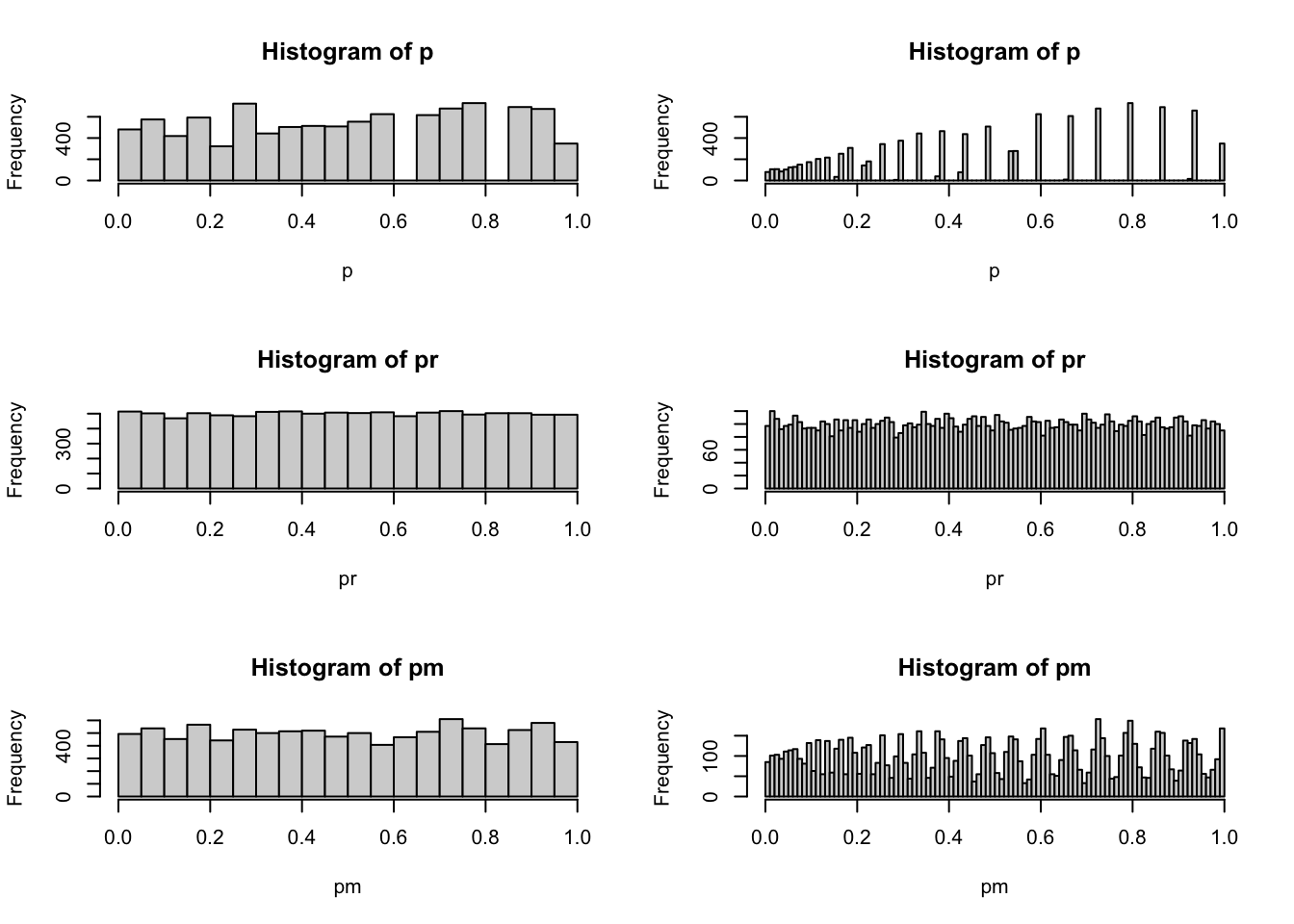这个结果非常有意思，第一个能看到的现象是如果数据本身存在规律性，那么p值的分布是一个离散分布。这个分布介于0到1之间，越接近0的部分越密集，越接近1的的部分越稀疏，但是如果计算小于0.05，0.5，0.9的比例情况，会发现这种稀疏分布依旧符合均匀分布的概率分布特征。如果数据不存在规律性，那么p值的分布就是很均匀的。如果数据混合了规律性与噪音，依然会显示出这种离散分布特征。下面我用qq图来观察下这个分布跟均匀分布的区别： set.seed(42) ref <- runif(10000) par(mfrow=c(1,1)) qqplot(ref,p)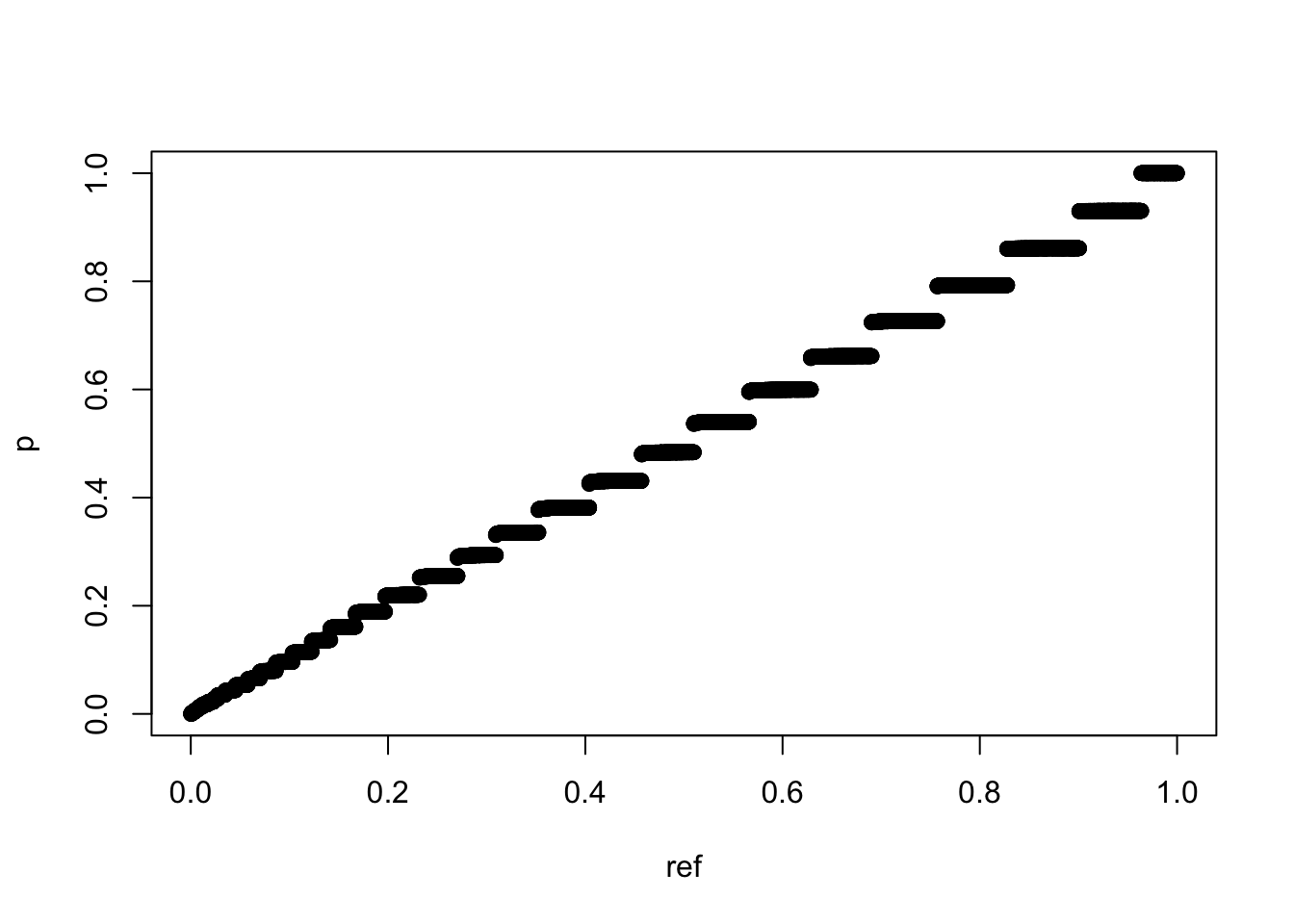qqplot(ref,pr)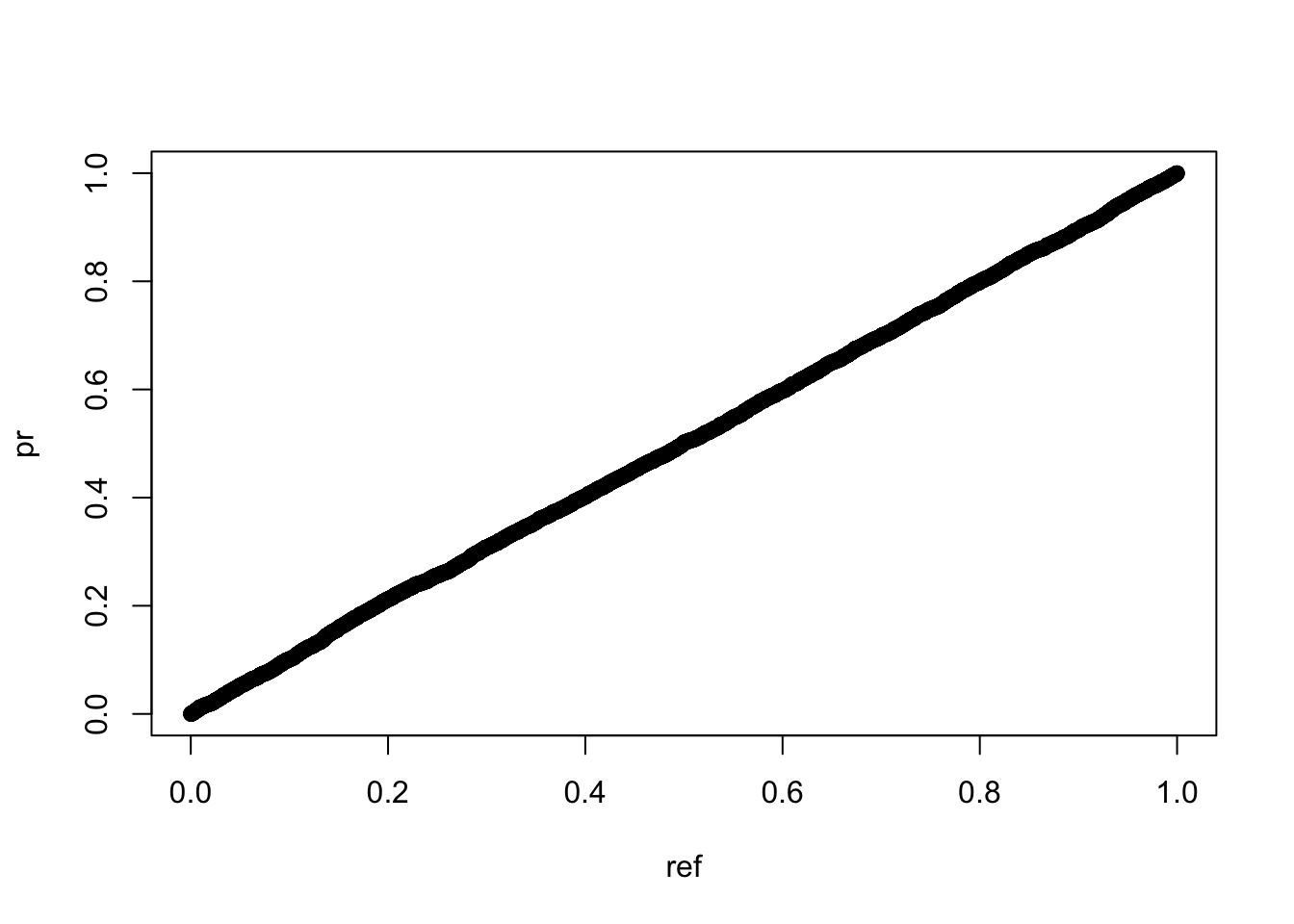qqplot(ref,pm)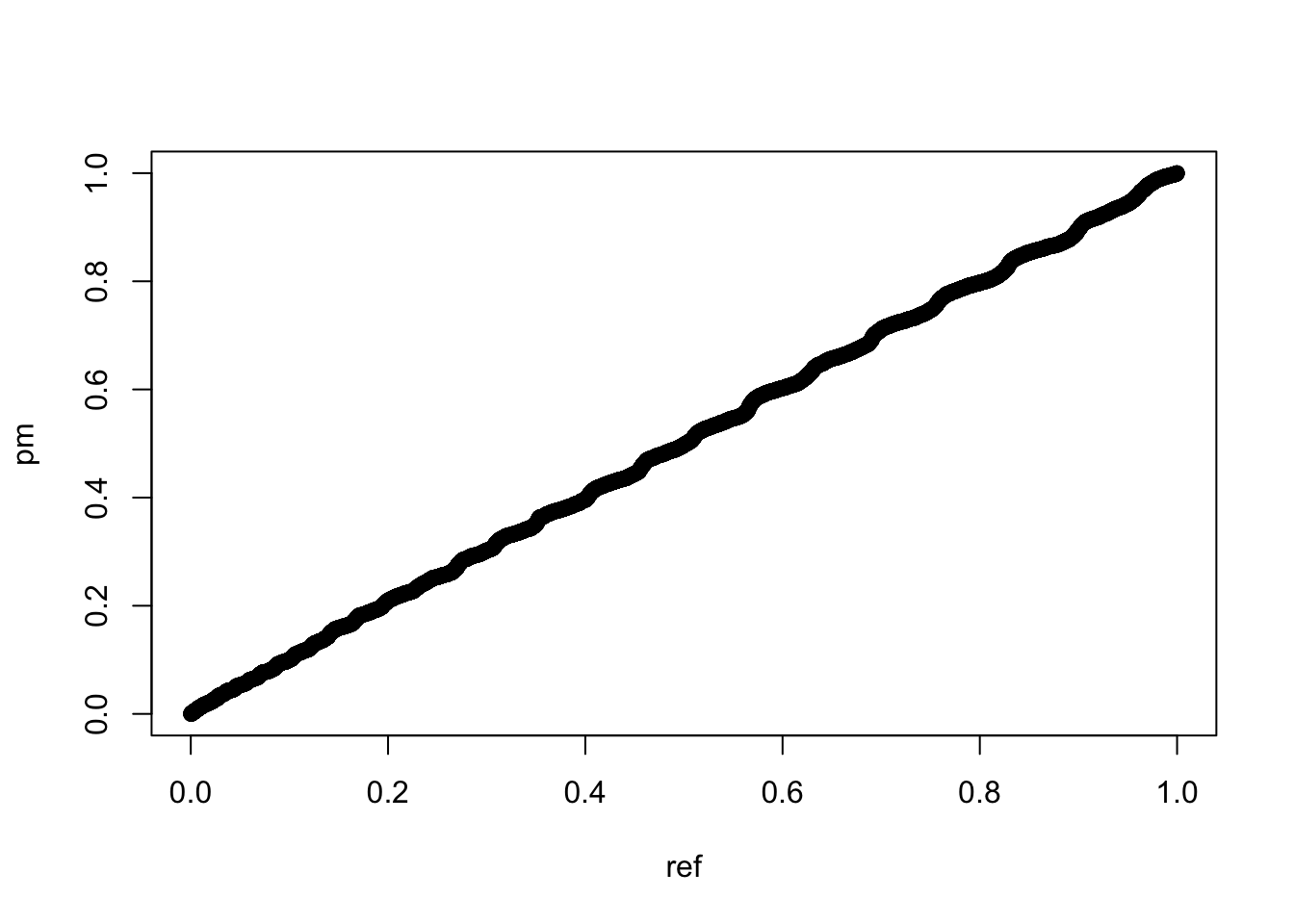可以看到，如果数据本身存在规律性，其随机化分组后的p值虽然跟均匀分布很接近，但qq图上确实会表现出前密后舒的螺旋延伸状态。 我虽然不清楚统计学上有没有对这个p值分布的研究，如果没有我先管它叫 MY Distribution ，谁让我名字缩写就是MY，这个“我的分布”可能对实验学科非常有意义。 这里为了区别我再做一个仿真，这次我不是对分组随机而是对采样随机： set.seed(1) # 固定分组 g <- factor(c(rep(1,260),rep(2,260))) p <- c() for(i in 1:10000){ # 随机化采样 x <- sample(c(rep(100,260),rep(200,260))+rnorm(520),520) p[i] <- t.test(x~g)$p.value
}
# 探索p值分布
sum(p<0.05)/length(p)
##  0.0406
sum(p<0.5)/length(p)
##  0.5344
sum(p<0.9)/length(p)
##  0.9356
hist(p,breaks = 20)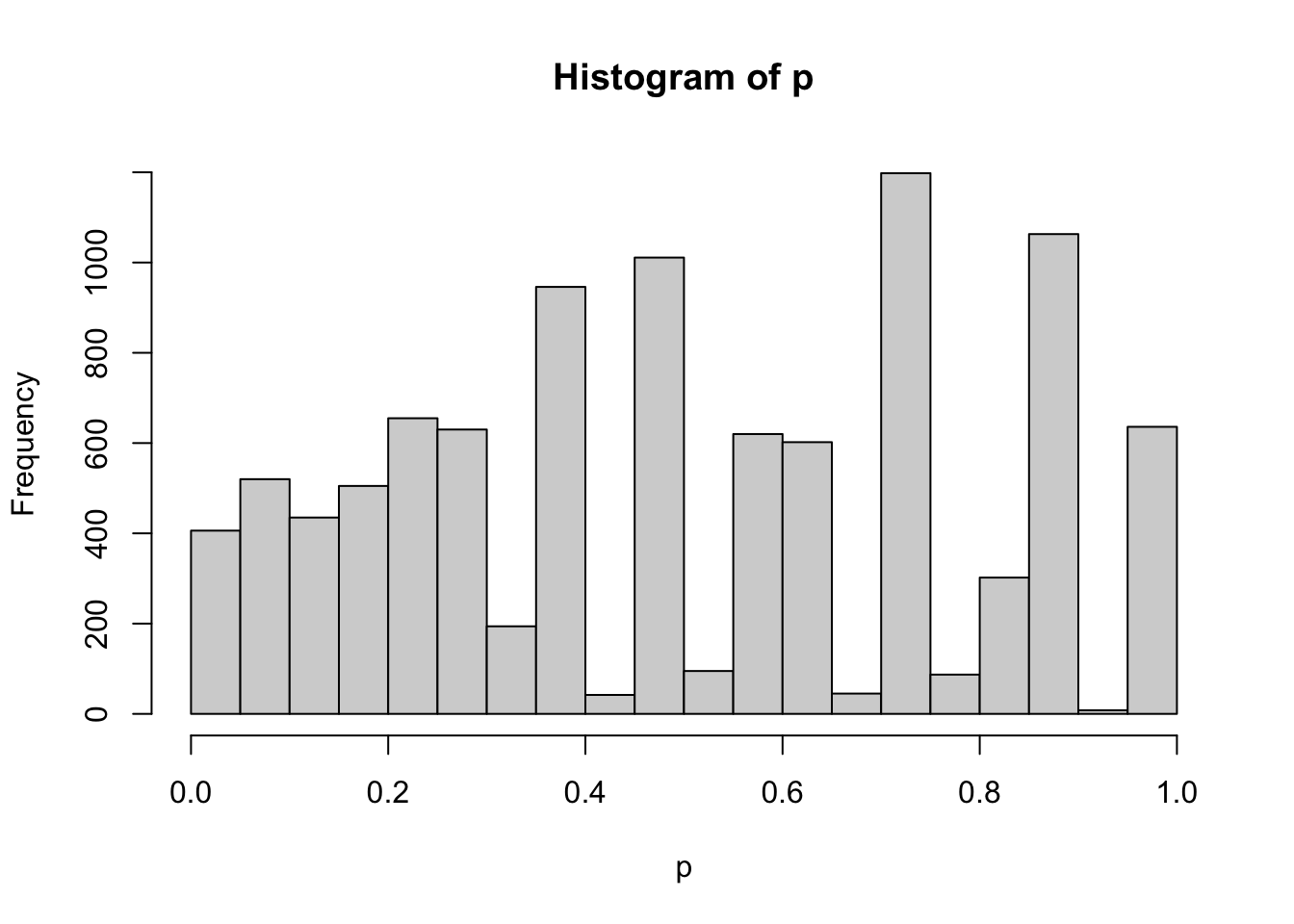hist(p,breaks = 100)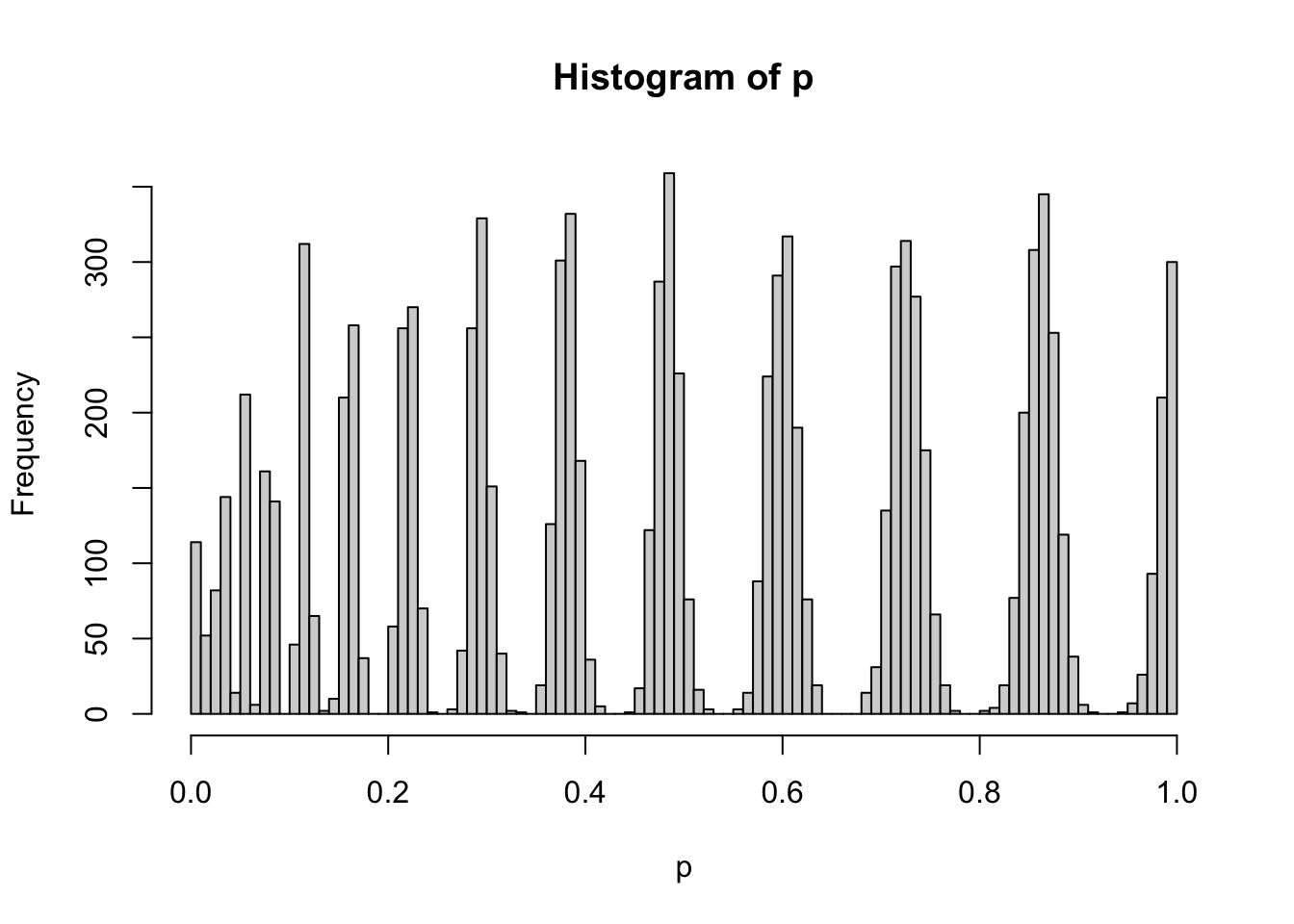qqplot(ref,p)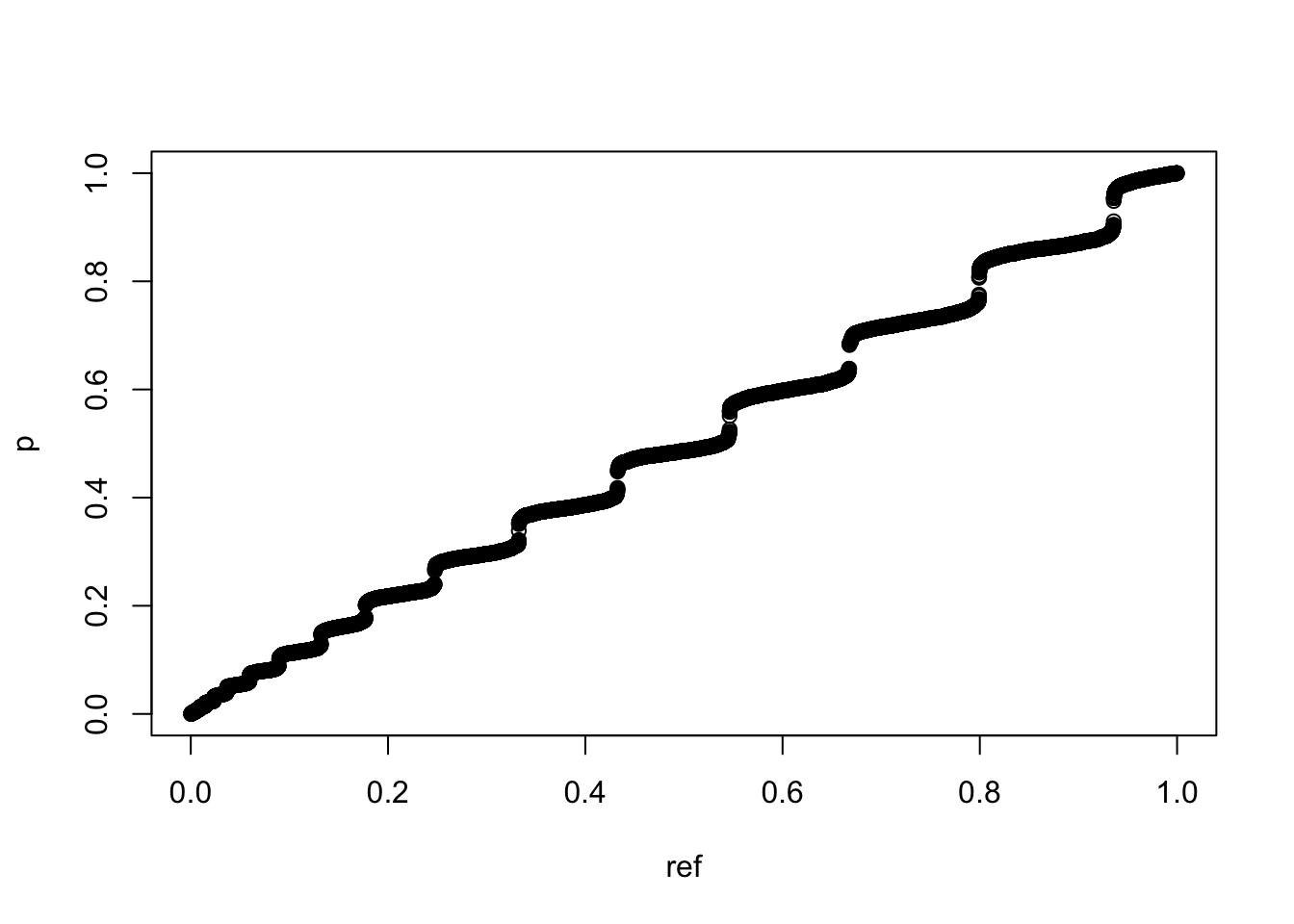set.seed(1)
pvalue <- NULL
for (i in 1:10000){
a <- rnorm(10,1)
b <- a+1
c <- t.test(a,b)
pvalue[i] <- c\$p.value
}
# 探索p值分布
hist(pvalue,breaks = 20)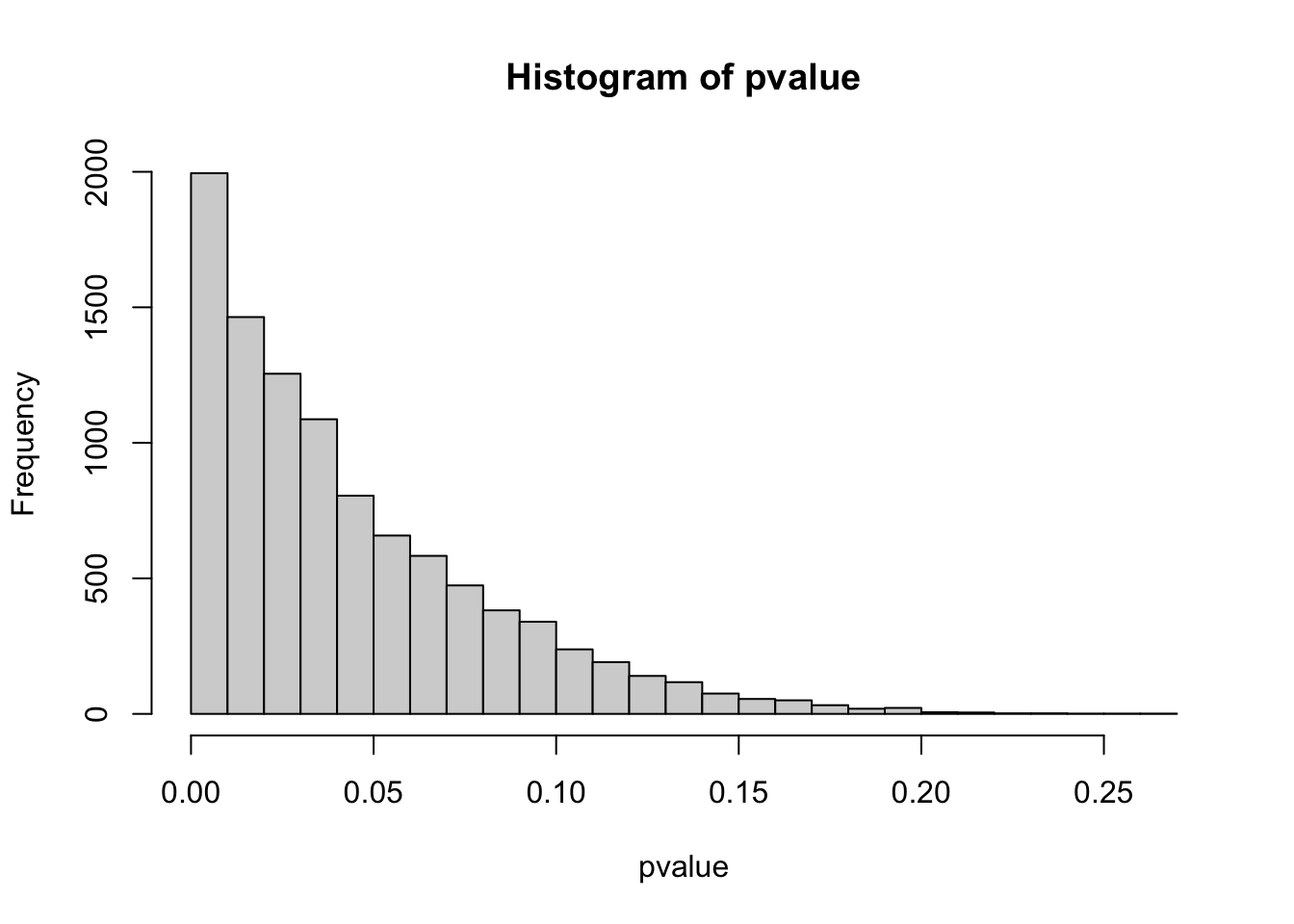hist(pvalue,breaks = 100)